Work

Work is energy that is transferred when an object is displaced by a force.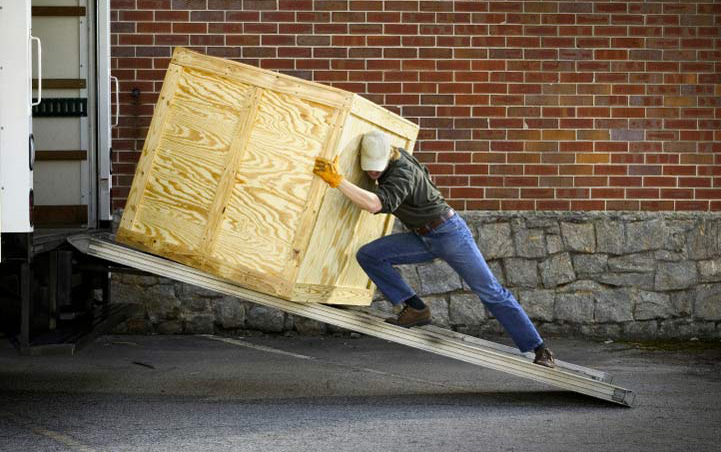The stronger the force and the farther the displacement, the more work is done. Since force and displacement are vectors, it also matters how much they are aligned.Work is defined as the dot product of force and displacement. Theta is defined as the angle between the force and displacement. Notice work is a scalar quantity, as we would expect, since it is transferred energy.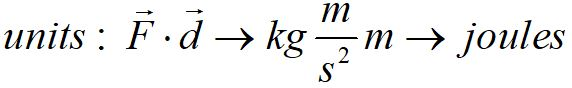The units of work are Newton-meters, equivalent to joules.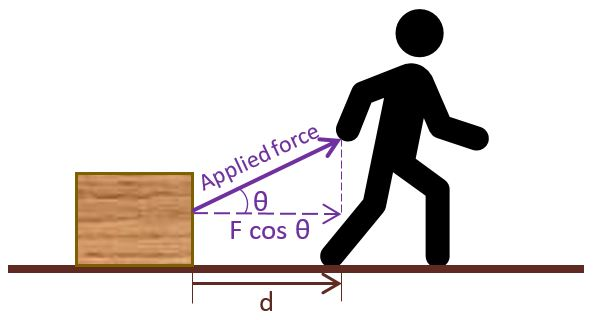As shown here, the work done by the applied force pulling the crate only includes the component of the force along the displacement of the crate.

Practice problems:

How much work is done by dragging a crate with a tension force of 10 Newtons at angle 30⁰ for a distance of 5 meters?

How high could you lift the crate, doing the same amount of work?

Transfer of energy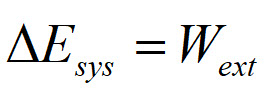When a process transfers energy into or out of a system by mechanical means, we say that work was done on or by the system. The change in energy of the system equals the external work done.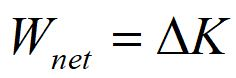The total work done by all of the forces on an object equal the change in kinetic energy of the object. This is known as the work-kinetic energy theorem.

Practice problem: An elevator of mass m starts from rest and accelerates upward with an acceleration a for a distance d. (use 10 m/s2 for g)

Assume:

m = 1000 kg

a = 1 m/s2

d = 20 m

How much work does the tension in the cable do on the elevator?

How much work is done by the gravitational force?

How fast is the elevator moving when it has gone the distance d?© 2018 - khadley.com

website developer Kathryn Hadley

web master: jay(at-sign)jirvinh.com